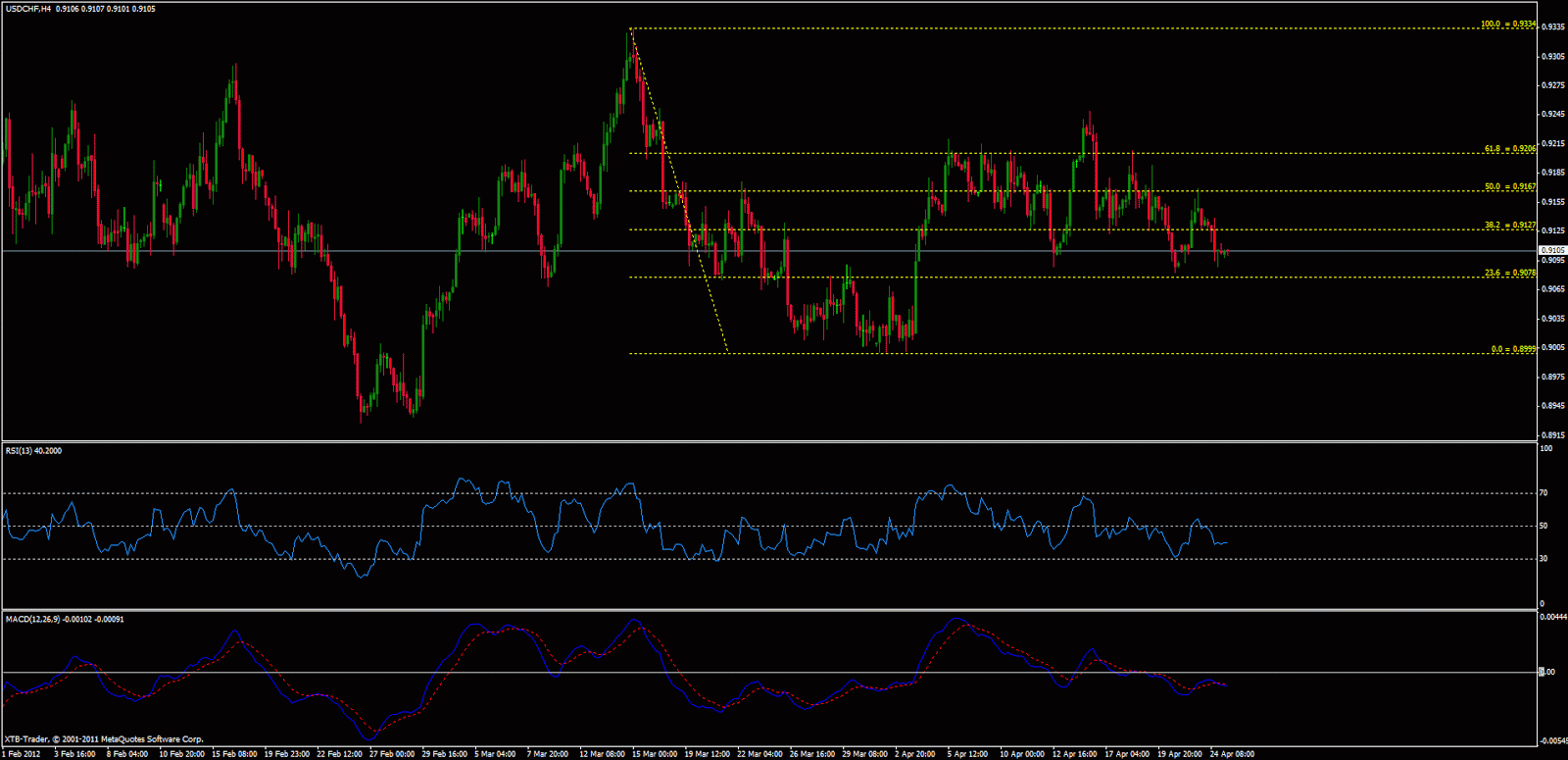# Fibonacci: As Tools

No comment 916 views
5 / 5 ( 1votes )

# Fibonacci: As ToolsFibonacci: As Tools

Fibonacci numbers and Fibonacci ratios are used by most traders to find potential levels of potential trend reversal in the market. As far back as the 1930s Ralph Elliott, who developed Elliott Wave Theory, notes that price movements have three basic characteristics: patterns, times, and ratios; all watching the Fibonacci sequences.

Indeed, the Fibonacci ratios have a big role in Elliott Wave Theory, but Fibonacci numbers and ratios are not only used by Elliott Wave, or Elliotticians, they are also used by other traders, especially to determine support and resistance levels and to identify potential trend change ( reversal) in the market.

The Fibonacci Summation series was introduced to the Western world by an Italian mathematician, Leonardo Pisano Bogollo, also known as Fibonacci (Bonacci’s son), in the 1202 Liber Abaci texts, although the series has been previously described in Indian mathematics.

This series is derived by searching for the sum of the two preceding numbers in the series, with 0 and 1 being the core number, or starting point, in the circuit. With 0 and 1 being the first two core numbers, the third number in the series is the sum of 0 and 1 (0 + 1), ie 1; the fourth number in the series is the number of the second and third numbers in the series, ie 1 + 1, and so on. Some of the first numbers in this series are:

0, 1, 1, 2, 3, 5, 8, 13, 21, 34, 55, 89, 144, 233, 377, 610, 987, … ∞

Series or series of numbers – these numbers will be the forerunner of Fibonacci gold ratio calculation.

Origin

The Fibonacci series appears in Indian mathematics, with respect to the prosodic of Sanskrit. In the Sanskrit tradition, there is an interest to enumerate all long syllable patterns (L) ie 2 units of duration, and short syllables (S) which is 1 unit of duration. Calculates L and S patterns that differ from the duration given in the Fibonacci numbers: the number of patterns consisting of long short syllables is the Fibonacci number Fm + 1.

Susantha Goonatilake writes that the development of the Fibonacci sequence “is partly due to Pingala (200 BC), then attributed to Virahanka (about 700 AD), Gopāla (about 1135), and Hemachandra (about 1150)”.Forex Signal 30 is the best forex system since 2009 and has been used by thousands of traders from around the world to generate profit in forex trading. This system is created by our team of Brilliant Forex Signal Team, this system is made as simple as possible for beginner and professional traders.